## Main

Sensitive imaging or detection of charges is an outstanding task in a variety of applications. The development, for example, of single-electron transistors (SET; ref. 5) has pushed charge sensing to an unprecedented sensitivity of 10−6 electron charge, and is being used in low-temperature detection and scanning applications2, and in sensors in quantum devices6. Inspired by the development of tunnelling microscopy a variety of scanning probes have been devised to measure surface electrical properties, such as scanning capacitance microscopy (SCM; ref. 7), scanning Kelvin probe (ref. 8) and electric field-sensitive atomic force microscopy (EFM; ref. 9). Indeed, the last method has shown the remarkable ability to detect the presence of individual charges 3.

We report on a fundamentally new method that uses the spin of single defect centres in diamond to sense electric-field-dependent shifts in energy levels. Sensitive electric-field detection is based on the remarkable properties of the NV centre10. The most notable of these are: the detection of fluorescence from single defects to provide an atom-sized local probe11, outstandingly long spin dephasing times12, as well as the controlled positioning of single centres 13,14. These properties have led to a variety of applications of the NV centre, ranging from quantum science15 and precision magnetic-field sensing16,17,18,19,20,21,22,23,24 to biolabelling25,26. It is the aim of the present work to explore the interplay between the Zeeman shift, local strain effects and the Stark shift of the ground state spin manifold and use the improved understanding of this interplay for the sensing of electric fields. We will show that the decoupling of the electric sensing transitions from magnetic fluctuations through careful alignment of the magnetic field provides a means to switch the sensor from ‘magnetic’ to ‘electric’ modes and plays a positive role in improving the spin decoherence time in a ‘dirty’ sensing environment. The rotation of the magnetic bias field provides the means to determine both the magnitude and three-dimensional orientation (up to a four-fold symmetry) of the electric field in the vicinity of single NV spins.

The NV centre is a well-studied defect in diamond, consisting of a substitutional nitrogen atom adjacent to a carbon vacancy (see Fig. 1a). The ground state spin triplet exhibits a zero-field splitting between the ms=0 and degenerate ms=±1 spin sub-levels of Dgs≈2.87 GHz. The spin state of the triplet can be polarized and read out via optical excitation to the excited triplet state, thereby enabling the implementation of optically detected magnetic resonance (ODMR) techniques using microwave sources tuned to the ground state zero-field splitting11. Stark shifts at low temperature have been demonstrated in the excited triplet states of single defect centres27 and the ground state of NV ensembles28. In our experiments, the electric field was generated by the application of a controlled voltage to a gold microstructure fabricated by lithography and electroplating directly on a bulk diamond sample containing NV centres. A particular NV centre close to the gold microstructure was identified and used for all of our measurements. The electric field at the location of the NV centre was calculated numerically for the given electrode geometry, taking into account the dielectric constant (ɛ=5.7) of the diamond (see Fig. 1b). To measure electric-field-induced shifts of spin sub-levels, we optically detected the electron spin-resonance transition between the ms=0 and ms=±1 sub-levels in the triplet ground state of the centre. On application of an electric field of about 3,000 V cm−1, resonance line shifts of 28.4 kHz were observed (the expected value is between 1 kHz for axial and 51 kHz for non-axial electric fields respectively). Before proceeding into the detailed description of the Stark effect in the ground state, we first analysed the spin Hamiltonian Hgs of the ground triplet state in the presence of magnetic, strain and electric fields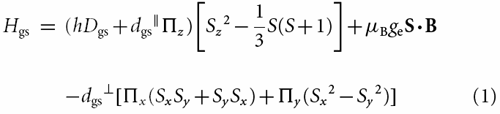Here dgs/h=0.35±0.02 Hz cm V−1 and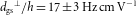(ref. 28) are the measured axial and non-axial components of the ground triplet state permanent electric dipole moment dgs,h is the Planck constant, μB is the Bohr magneton, ge is the electron g -factor, B is the applied magnetic field, and S is the electron spin operator. Note that the coordinate system is defined as depicted in Fig. 2a (that is, the z axis is parallel to the axial symmetry axis of the NV centre which connects the nitrogen and vacancy sites).

It is worth mentioning that dgs is much smaller than the excited state des because of the antisymmetric combination of molecular ex, ey type orbitals in the ground state. Consequently, dgs is non-zero only because of spin orbit coupling between the ground and excited spin triplet states, such that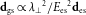, where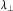is the centre’s non-axial spin orbit parameter and Ees is the optical energy difference between the triplet states (see Supplementary Information). Given that crystal strain can be treated effectively as a local static electric field σ (ref. 27), it combines with the applied electric field E to give the total effective electric field Π=E+σ at the NV centre. In the regime where DgsμBgeB and DgsdgsΠ, second-order perturbation theory can be applied to obtain the approximate energy solutions of Hgs. Considering fixed magnetic and strain fields, the change in the magnetic transition frequency Δω± between the ms=0 and the ms=±1 spin sub-levels caused by the application of an electric field is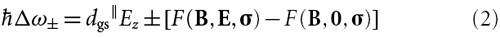where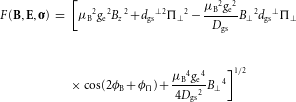and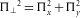,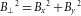, tanϕΠyx, and tanϕB=By/Bx. Given that the Zeeman effect is much stronger than the Stark effect in the ground state, the first-order appearance of μB2ge2Bz2 in F indicates that for the expected case, dgsΠ/μBgeB1, careful alignment of the magnetic field in the non-axial plane is required, otherwise the detectable change in the transition frequency caused by an electric field reduces to ΔωdgsEz, which, as dgs is small, severely reduces the detectable electric field strength. This argument also indicates that the presence of non-axial strain reduces the dominating effect of axial magnetic fields on F by increasing the ratio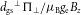(see Supplementary Information). This is important for future sensing applications using nanodiamonds. These typically possess a strain of several tens of MHz, which is larger than the Zeeman shift due to the Earth magnetic field (2 MHz), such that sensing without additional magnetic field alignment seems feasible. Furthermore, a zero magnetic field environment can be reached using a magnetically shielded set-up, which further aids future biological sensing applications.

The dependence of F on the non-axial magnetic field orientation ϕB indicates that the orientation of the electric field will be determined up to a four-fold symmetry if Δω is measured for several magnetic field orientations. Figure 2c shows the theoretical polar plot of Δω as a function of ϕB for the special case (solid blue line) where the effective strain and applied electric fields are in parallel directions or negligible strain exists. As Δω depends on both the orientations of the effective strain and applied electric fields, the symmetric ‘four-leaf’ pattern of the aligned case will distort and rotate if the applied electric field is rotated with respect to the strain field (purple dashed line). If the strain field is characterized for a given NV centre, the measurement of the Δω polar pattern and subsequent analysis using equation (2) allows the applied electric field magnitude and orientation to be determined.

Indeed the measured polar plot of Δω as a function of ϕB (Fig. 2d) agrees very well with the theoretical analysis. The small differences between theory and the measurements may be explained by a small axial magnetic field uncertainty of ±5 μT that was present. The rotation and distortion of the polar pattern from the symmetric ‘four-leaf’ pattern indicates that the applied electric and effective strain fields are not aligned. A least square fit of the polar pattern yields the field parameters: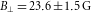, dgsEz/h=−4.2±2.1 kHz,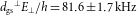, ϕE=32°±1°, and ϕσ=22°±9°. Note that the non-axial orientations of the electric ϕE and strain ϕσ fields are taken with respect to the laboratory reference frame. The observed strong decay of the electric-field-induced shift Δω with increasing axial magnetic field strength (depicted in Fig. 2b) also agrees well with the theoretical model and reinforces the requirement for precise alignment of the magnetic field in the non-axial plane. The measurements of Fig. 2b and d and the underpinning theoretical analysis clearly demonstrate how the combination of ODMR techniques and precise magnetic field alignment and manipulation can be used to detect the vector electric field.

As in magnetic-field sensing18, electric fields are most sensitively detected when a field-induced phase accumulation is used. As an example, Fig. 3c shows the amplitude of a Hahn echo cos(Δω τ) as a function of electric field strength recorded by a two pulse spin echo sequence with a fixed free evolution time τ. The sinusoidal modulation of the measured echo intensity on the increase of the electric field allows a precise determination of the minimally detected electric field, δ Emin, which can be expressed as the ratio of the standard deviation of a Hahn signal measurement σsn and the gradient of the oscillation of the Hahn signal dS=δ S/δ E (as indicated in Fig. 3c)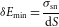In our measurement, σsn depends on the photon shot noise, enabling a photon shot-noise limited measurement, as demonstrated in Fig. 3b.

As the amplitude of the Hahn signal decays with τ owing to decoherence, δ S likewise decays with τ. τ itself is limited by the dephasing time T2. Consequently, an optimal τ exists that maximizes the sensitivity, and this was determined for the Hahn echo (a.c. electric field) and the free induction decay (FID) (d.c. electric field) measurements. The optimal τ for both cases are included with the plot of δ Emin in Fig. 3b. For a.c. (d.c.) electric-field sensing, a sensitivity of 202±6 V cm−1 Hz−1/2(891±21 V cm−1 Hz−1/2) was achieved, with the smallest detected field being 7.5 V cm−1(173 V cm−1). Here we use the standard definition of the bandwidth as the inverse of twice the measurement time. The intricate combined interaction of strain, electric and magnetic fields that enables this electric-field sensing technique indicates that two different sensing regimes exist, each suited to different sensing applications (see Supplementary Information). One regime is characterized by a non-axial magnetic field that dominates over the strain, that is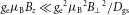,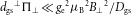and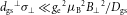, and can be engineered by precise magnetic field alignment (as performed in this work) and in which the electric-field sensitivity is independent of the electric, magnetic and strain field strengths. The second regime is characterized by zero magnetic field and a natural strain that is large compared to the electric field shift, such as the strain that occurs in nanodiamonds. In this case, the energy level shift encodes the projection of the electric field onto the major strain direction. Both regimes are suitable for vector electric field measurements.The principal factors that govern the sensitivity of this technique are the T2* and T2 of the NV centre. As the measured T2 (304±36 μs) of the centre investigated was rather short for an NV centre in bulk diamond, an improvement in the measured sensitivity is expected if an NV centre in a higher purity sample was used. In addition, as T2 values—similar to the one used here—are now also found for shallow implanted defects29, the method demonstrated here is suitable for charge sensing applications. The sensing scheme presented here exploits the zero axial magnetic field limit of the Hamiltonian where the electrically sensitive transitions are decoupled from magnetic fluctuations (see subsequent paragraph), as is seen most strikingly by the observed narrow line widths in Fig. 1c. Critically, in addition to allowing the nanosensor to be switched from magnetic- to electric-field sensing, it also indicates that decoherence due to impurity spins could be overcome, even in a ‘dirty’ environment, and holds promise for a powerful, yet material-independent route towards quantum metrology under biological conditions.

Figure 3d demonstrates that the observed T2* strongly depends on the applied axial magnetic field component and reaches a maximum when the applied axial magnetic field component is zero. This dependence of T2* on the magnetic field is best understood by analysing the eigenstates of the spin Hamiltonian (1) in the absence of applied electric and magnetic fields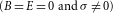. In this case, the eigenstates of (1), expressed in terms of the eigenstates of Sz{|0〉,|+1〉,|−1〉}, are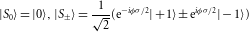and have energies E0=−(2/3)(h Dgs+dgsσz) and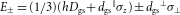respectively. The expectation value of the spin operator 〈S〉 is zero for each of these eigenstates, which implies that, as long as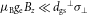, there will be no first order Zeeman shift of the energies due to a magnetic field. However, the expectation value of the electric dipole operator 〈dgs〉 is maximal. Therefore, in this regime, decoherence of the ground state spin will be dominated by electric and strain noise and will be minimally affected by magnetic noise. The opposite is true if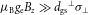, in which case the eigenstates and energies become approximately {|0〉,|+1〉,|−1〉} and E0=−(2/3)Dgs and E±=(1/3)Dgs±μBgeBz respectively. The application of an axial magnetic field thereby switches the decoherence of the ground state spin from being dominated by electric and strain noise to being dominated by magnetic noise. For the intermediate case,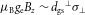, it is expected that the decoherence will be influenced by all sources of noise.

In summary, harnessing the interplay between strain, electric and magnetic fields, the ground state spin of single NV centres has been used for vector electric-field sensing on the nanoscale. A photon shot-noise limited a.c. electric-field sensitivity of 202±6 V cm−1 Hz−1/2 was achieved, allowing the detection of the electrostatic field produced by a single elementary charge located at a distance of 150 nm from the sensing NV spin within one second of averaging. An electric field strength as small as 14 V cm−1 was measured on averaging for about 100 s. This is roughly equivalent to sensing a single electron charge from a distance of 35 nm with a signal to noise ratio of more than 1,000. NVs as close as 40 nm to the surface show coherence times of the order of 100 μs (ref. 29), therefore single external charge detection with the NV centre is within reach (the sensitivity is expected to be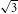lower for T2100 μs). Tailoring the material aspects of diamond12,29, efficient photon collection30 and multipulse control schemes20,21,31 are options available for further improvement.

Benchmarking the achieved sensitivity against other precision charge measuring techniques, such as SET, SCM, EFM and Kelvin probe microscopy, shows that the sensitivity of the method presented here is approximately two orders of magnitude less than the most sensitive electric-field detection technique, this being the SET at a standoff distance of 100 nm. However, the key advantage of the NV centre is the fact that it is an atomic scale sensor and therefore can potentially perform precision sensing at distances as close as a few nanometres under ambient conditions. The first steps towards NV sensing on the nanometre scale under ambient conditions have already been reported32. This regime of applicability is in contrast to all of the above mentioned techniques, in terms of both spatial resolution and operating conditions, and opens up the possibility to image individual charges with nanometre spatial resolution under ambient conditions. In addition, the NV sensor has the unique ability to switch between electric- or magnetic-field detection modes, making it a universal detector system with unprecedented versatility.

## Methods

Magnetic field control was realized using two Helmholtz coil pairs for the x and y axes and a single coil for the z axis. The resonant microwave field was generated by a set of waveguides fabricated as part of the gold microstructure. The diamond sample used was a CVD grown diamond with a low nitrogen content and natural abundance of 13C impurity spins (electronic grade Element 6).

To accurately align the magnetic field in the non-axial plane (Bz=0), the orientation-dependent Zeeman splittings of the NV centre’s ground state hyperfine structure were observed using CW ODMR techniques. The hyperfine interaction of the NV centre’s electronic spin with the14N nuclear spin results in three observable magnetic transition frequencies separated by 2.2 MHz. Each magnetic transition corresponds to a change in the electronic spin projection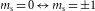, but conserves nuclear spin projection, such that the low and high frequency transitions are between hyperfine states with mI=±1 and the central frequency transition is between hyperfine states with mI=0. For magnetic fields applied to NV centres with a crystal strain that satisfies dgsσ/μBgeB1, the hyperfine states with mI=±1 are not split by the presence of strain and/or a non-axial magnetic field, but are split by the axial component of the magnetic field. Consequently, the magnetic field can be aligned in the non-axial plane by manipulating the field and observing the pulsed CW ODMR spectra such that there is no splitting in either the low or high frequency hyperfine magnetic resonance lines. This alignment technique is limited by the linewidth of these hyperfine lines, but the axial magnetic field component can be determined to a particular uncertainty by interpolating the observed magnetic fields in relation to the coil currents. Once aligned, the magnitude of the non-axial strain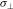can be inferred from the splitting of the central line as long as the non-axial magnetic field strength is known, and for the NV centre studied it was found to be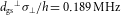. Given that only the central hyperfine transition, which involves states with mI=0, is susceptible to splitting by strain and electric fields, it was this transition that was used in the electric field measurements, reducing the observable ODMR contrast to a third.

Given that the ground triplet state is an orbital singlet and the Stark effect is purely an orbital interaction, the presence of the effect in the ground triplet state is forbidden without some prior mixing of the orbital and spin components of the centre’s electronic states. However, the effect is allowed without such mixing in the excited triplet state, as it is an orbital doublet. Indeed, the strong linear splitting of the orbitally degenerate ms=0 spin sub-levels of the excited triplet has been well documented and attributed to the presence of a permanent electric dipole moment des (ref. 27). The application of the well-established molecular model yields des=〈ex|x|ex〉+〈ex|y|ey〉+〈a1|z|a1〉+3〈ex|z|ex〉, where a1, ex and ey are molecular orbitals that are constructed from the dangling s p3 atomic orbitals of the neighbouring carbon atoms. Note that the NV defect has C3v symmetry and the orbitals are of symmetry type A1 and E, respectively. The charge distributions exex and exey, responsible for the dominant non-axial components of des, are shown in Supplementary Fig. S1. The previous observation of the weak linear splitting of the degenerate ms=±1 spin sub-levels of the ground triplet state indicates that the Stark effect in the ground triplet state is indeed a consequence of a higher-order interaction. Spin–orbit interaction is the immediately obvious higher-order interaction capable of the required mixing, and the application of the molecular model indicates that the ms=±1 spin sub-levels of the ground triplet state are mixed with the degenerate ms=0 spin sub-levels of the excited triplet state by the non-axial components of spin–orbit interaction. This mixing between the ground and excited triplet states explains the existence of the weaker linear Stark effect in the ground triplet state, as the permanent electric dipole moment of the ground triplet state, dgs, is related to the dipole moment of the excited triplet state by the square of the non-axial spin–orbit interaction strength, such that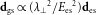, where Ees is the energy of the excited triplet state.